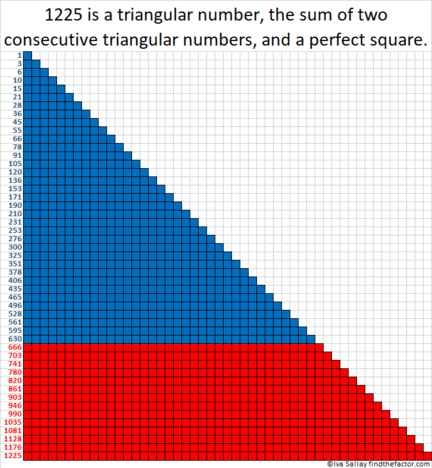# 1225 is a Triangular Number, a Perfect Square, and . . .

1 and 36 are both triangular numbers and square numbers. The next number that can make the same claim is 1225. Why is this so?

1225 is a triangular number that just happens to be the sum of two consecutive triangular numbers. Two consecutive triangular numbers can always be made into a perfect square. Here’s a gif that tries to illustrate these facts about 1225:GIFs like this at MakeaGif

1225 is the 49th triangular number because 49(50)/2 =1225

It is the 35th square number because 35² = 1225

That it is both a triangular number AND a square number is pretty remarkable. But guess what? 1225 is ALSO a hexagonal number.

1225 is the 25th hexagonal number because (25)(2·25 – 1)= 1225
In fact, 1225 is the smallest number greater than 1 that is a triangle, a square, AND a hexagon! (Yeah, 1 is also all three and a whole more, but does 1 dot REALLY look like a triangle, a square, a hexagon and everything else all at the same time?)
All of these facts are great reasons to get very excited about the number 1225. Here are some more facts about this number:
• 1225 is a composite number.
• Prime factorization: 1225 = 5 × 5 × 7 × 7, which can be written 1225 = 5²× 7²
• The exponents in the prime factorization are 2 and 2. Adding one to each and multiplying we get (2 + 1)(2 + 1) = 3 × 3 = 9. Therefore 1225 has exactly 9 factors.
• Factors of 1225: 1, 5, 7, 25, 35, 49, 175, 245, 1225
• Factor pairs: 1225 = 1 × 1225, 5 × 245, 7 × 175, 25 × 49, or 35 × 35
• 1225 is a perfect square. √1225 = 35
Not only is 1225 a triangular number that is the sum of two other triangular numbers, but
1225 is also a square that is the sum of two other squares!
28² + 21² = 35² = 1225

1225 is the hypotenuse of two Pythagorean triples:
735-980-1225 which is (3-4-5) times 245
343-1176-1225 calculated from 28² – 21², 2(28)(21), 28² + 21²,
but it is also (7-24-25) times 49

1225 looks like a square in some other bases:
It’s 441 in BASE 17,
169 in BASE 32,
144 in BASE 33,
121 in BASE 34, and
100 in BASE 35

OEIS.org reports that 1225 is the smallest number that can be written as the sum of four cubes three different ways. It looks like these are the three ways:
10³ + 6 ³ + 2³ + 1³ = 1225
9³ + 6³ + 6³ + 4³ = 1225
8³ + 7³ + 7³ + 3³ = 1225

1225 is, indeed, the smallest number that has all the special properties listed above!

This site uses Akismet to reduce spam. Learn how your comment data is processed.